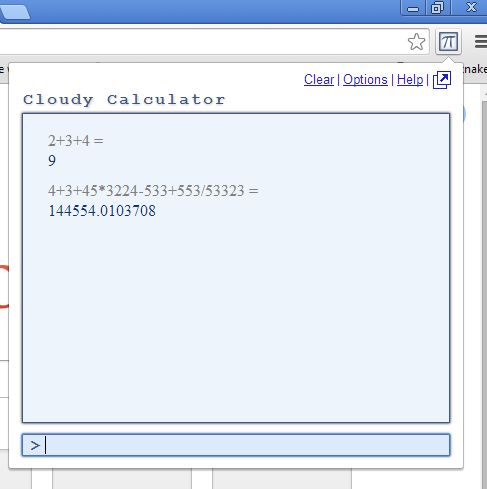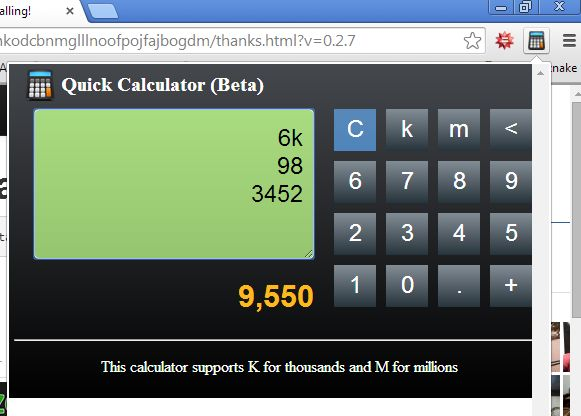Editor Ratings:
User Ratings:
[Total: 0 Average: 0]

Here’s a list of 5 math extensions for Google Chrome which you can use for everything from helping your kids learn math while they’re using their favorite web browser to performing advanced mathematical calculations yourself. We mentioned helping kids learn math, but actually some of the extensions that we talked about here can be of assistance to more advanced mathematicians.

It doesn’t matter if you’re in middle school, high school or college, these extensions can only help you out get better grades and pass that un-passable exam that’s been giving you headaches at school.

##(adsbygoogle = window.adsbygoogle || []).push({});

We’re gonna start things off with one of those math extensions that we said is aimed at slightly more advanced users.

Math Anywhere is gonna take Latex and MathML syntax off of websites and convert them into actual mathematical formulas. With it, instead of looking at incoherent Latex code, you’ll be able to see what kind of math formulas and expressions they represent. To turn on the conversion on a certain website just left click on the extension icon.

Get Math Anywhere.

##With this extension you get access to not just one, but over a dozen different mathematical and logical games.

Everything from simple addition and subtraction, to more advanced puzzles that are aimed more at sharpening logical reasoning are available. Your kids will love them, even if they might not be that crazy about math.

##(adsbygoogle = window.adsbygoogle || []).push({});

You can’t be a mathematician without having a calculator extension installed into Chrome.

Cloudy Calculator is exactly that. With it you can easily perform advanced calculations and if by some chance it doesn’t know the answer to a math problem that you type into it, it’s gonna turn to Google and its famous in-search calculator for help. To solve a problem just type into the field at the bottom and hit the return key. Results are gonna be showed in the large box that takes up most of the extension. Problems can be typed using the standard math syntax, square root is for example, “sqrt4”.

##Let’s not forget about another super important tool for any would be mathematician, the Wolfram Alpha website.

On the Wolfram Alpha website you can perform various mathematical calculations. From super simple to super advanced. This is the official extension for it that’s gonna let you quickly type in math expressions into it and then when you click the return key it will take you to the Wolfram Alpha website for the results.

##Seeing how they are important thing to have if you’re a mathematician, we decided to add another calculator extension to the mix.

This one isn’t as advanced as the Cloudy Calculator, but if you need to add, subtract, multiply or divide something quickly and on the go, this math extension is just the thing you need.

Get Quick Calculator.

## Conclusion

We were impressed the most with Math Anywhere and Cloudy Calculator, but other extensions are also very useful. If you have a little kid, don’t forget the awesome collection of math games that the Play Math Games Online extension gives you access to.

 Editor Ratings: User Ratings:[Total: 0 Average: 0] Works With: Google Chrome Free/Paid: Free